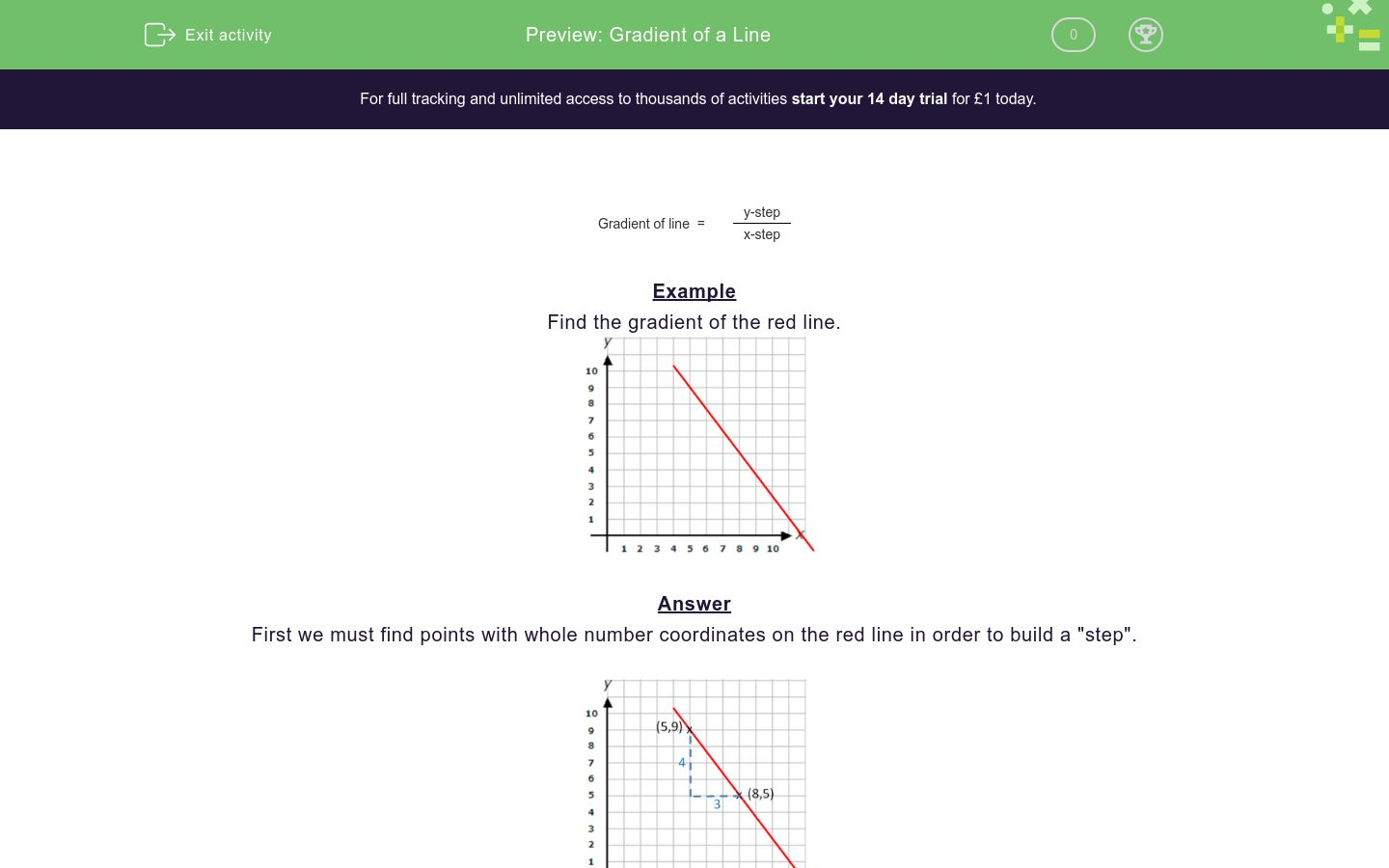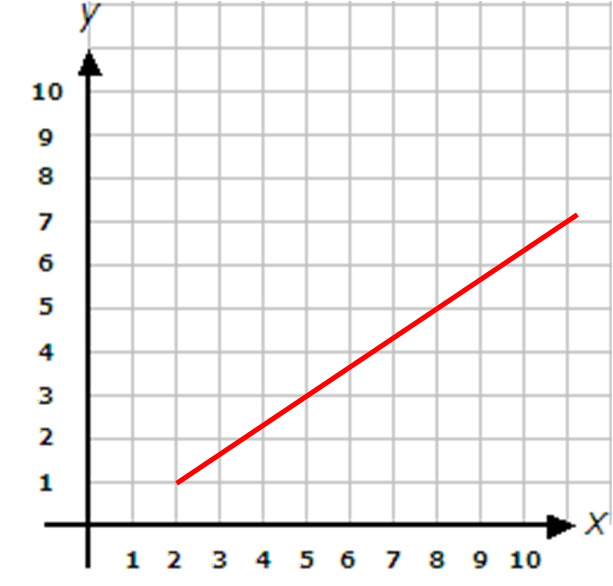### Affordable pricing from £10/month

In this worksheet, students work out the gradient of a line on coordinate axes.Key stage:  KS 4

Curriculum topic:  Algebra

Curriculum subtopic:  Use y = mx + c to Identify Parallel and Perpendicular Lines

Difficulty level:### QUESTION 1 of 10

 Gradient of line  = y-step x-step

Example

Find the gradient of the red line.First we must find points with whole number coordinates on the red line in order to build a "step".The line slopes from top left to bottom right so the gradient will be negative.

The vertical part of the step (y-step) = -4

The horizontal part of the step (x-step) = 3

 Gradient of line  = y-step = -4 x-step 3

Find the gradient of the red line.

(If it is not a whole number, write it as a fraction in the form a/b)Find the gradient of the red line.

(If it is not a whole number, write it as a fraction in the form a/b)Find the gradient of the red line.

(If it is not a whole number, write it as a fraction in the form a/b)Find the gradient of the red line.

(If it is not a whole number, write it as a fraction in the form a/b)Find the gradient of the red line.

(If it is not a whole number, write it as a fraction in the form a/b)Find the gradient of the red line.

(If it is not a whole number, write it as a fraction in the form a/b)Find the gradient of the red line.

(If it is not a whole number, write it as a fraction in the form a/b)Find the gradient of the red line.

(If it is not a whole number, write it as a fraction in the form a/b)Find the gradient of the red line.

(If it is not a whole number, write it as a fraction in the form a/b)Find the gradient of the red line.

(If it is not a whole number, write it as a fraction in the form a/b)• Question 1

Find the gradient of the red line.

(If it is not a whole number, write it as a fraction in the form a/b)2
• Question 2

Find the gradient of the red line.

(If it is not a whole number, write it as a fraction in the form a/b)-1/2
• Question 3

Find the gradient of the red line.

(If it is not a whole number, write it as a fraction in the form a/b)2/3
• Question 4

Find the gradient of the red line.

(If it is not a whole number, write it as a fraction in the form a/b)-1
• Question 5

Find the gradient of the red line.

(If it is not a whole number, write it as a fraction in the form a/b)1/3
• Question 6

Find the gradient of the red line.

(If it is not a whole number, write it as a fraction in the form a/b)2/3
• Question 7

Find the gradient of the red line.

(If it is not a whole number, write it as a fraction in the form a/b)-2
• Question 8

Find the gradient of the red line.

(If it is not a whole number, write it as a fraction in the form a/b)-4
• Question 9

Find the gradient of the red line.

(If it is not a whole number, write it as a fraction in the form a/b)1/4
• Question 10

Find the gradient of the red line.

(If it is not a whole number, write it as a fraction in the form a/b)